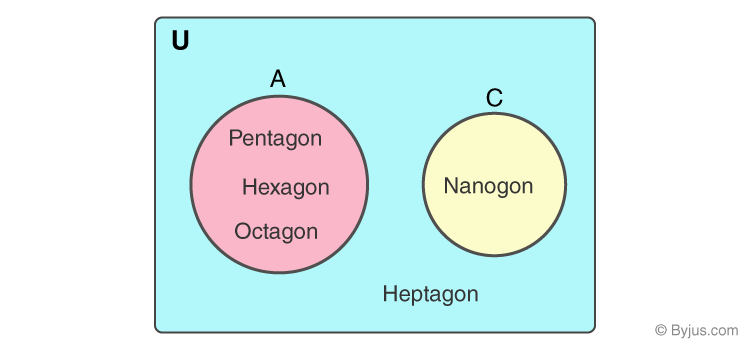# Universal Set

A universal set (usually denoted by U) is a set which has elements of all the related sets, without any repetition of elements. Say if A and B are two sets, such as A = {1,2,3} and B = {1,a,b,c}, then the universal set associated with these two sets is given by U = {1,2,3,a,b,c}.

In Mathematics, the collection of elements or group of objects is called a Set. There are various types of sets like Empty set, Finite set, Infinite set, Equivalent set, Subset, Superset and Universal set. All these sets have their own importance in Mathematics. There is a lot of usage of sets in our day-to-day life, but normally they are used to represent bulk data or collection of data in a database. For example, our hand is a set of fingers, where each finger is different from the other one. The notation of set is usually given by curly brackets, {} and each element in the set is separated by commas like {4, 7, 9}, where 4, 7, and 9 are the elements of sets.

Universal set contains a group of objects or elements which are available in all the sets and is represented in a Venn diagram

## Universal Set Definition

A universal set is a set which contains all the elements or objects of other sets, including its own elements. It is usually denoted by the symbol ‘U’.

Suppose Set A consists of all even numbers such that, A = {2, 4, 6, 8, 10, …} and set B consists of all odd numbers, such that, B = {1, 3, 5, 7, 9, …}. The universal set U consists of all natural numbers, such that, U = {1, 2, 3, 4, 5, 6, 7, 8, 9, 10,….}. Therefore, as we know, all the even and odd numbers are a part of natural numbers. Therefore, Set U has all the elements of Set A and Set B.

There is no formula to find the universal set, we just have to represent all the elements in a single which is collectively called a universal set.

Fact: There is no standard notation for Universal set symbol, it can also be denoted by any other entity like ‘V’ or ‘ξ’.

 Example: Let us say, there are three sets named as A, B and C. The elements of all sets A, B and C is defined as; A={1,3,6,8} B={2,3,4,5} C={5,8,9} Find the universal set for all the three sets A, B and C. Answer: By the definition we know, the universal set includes all the elements of the given sets. Therefore, Universal set for sets A, B and C will be, U={1,2,3,4,5,6,8,9} Explanation: From the above example, we can see that the elements of sets A, B and C are altogether available in Universal set ‘U’. Also, if you observe, no elements in the universal set are repeated and all the elements are unique.

Note: If Universal set contains Sets A, B and C, then these sets are also called subsets of Universal set. Denoted by;

A ⊂ U (A subset of U)

B ⊂ U (B subset of U)

C ⊂ U (C subset of U)

## Venn Diagram of Universal Set

For Venn diagram representation of the universal set, we can take the example as;

U={heptagon} consisting of set A={pentagon, hexagon, octagon} and set C={nonagon}.We can understand the concept of Universal set also by taking an example of the real world. In this world, we have set of a human being, set of animals and also set of all living things, which we can consider as a subset of U. But we cannot consider a set of trees as a subset of U.

### Complement of Universal Set

There is a complement of set for every set. The empty set is defined as the complement of the universal set. That means where Universal set consists of a set of all elements, the empty set contains no elements of the subsets. The empty set is also called a Null set and is denoted by ‘{}’.

## Universal set and Union of set

Students sometimes get confused between universal set and union of the set. They think both are the same but that is not true.

The universal set is a set which consists of all the elements or objects, including its own elements. It is represented by just a symbol ‘U’. But the union of sets is an operation performed on two sets, say A and B, which results in a set that has all elements belonging either to set A and set B or both. The operand of union of set is given by ‘∪’.

For example, Set A = {a,b,c} and set B={c,d,e} and U={1,2}. Therefore, the universal set for set A, B and U itself will be;

U ={a,b,c,d,e,1,2}

You can see, U is also a set which collectively shows all the elements of A, B and of itself.

Now, the union of set A and B is given by:

A∪B = {a,b,c,d,e}

## Video Lesson on What are Sets